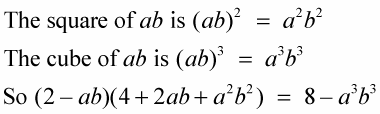##### Flipping Houses For DummiesAn expression that results in the difference between two cubes is usually pretty hard to spot. The difference of two cubes is equal to the difference of their cube roots times a trinomial, which contains the squares of the cube roots and the opposite of the product of the cube roots.

To recognize what distribution results in the difference of two cubes, look to see if the distribution has a binomial, (ab), which is the difference between two terms, times a trinomial,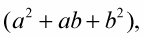which has the squares of the two terms and the opposite of their product. For example,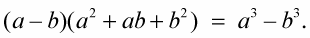A number’s opposite is that same number with a different sign in front. If the number is a negative number, then its opposite would be positive, and vice versa.

Example: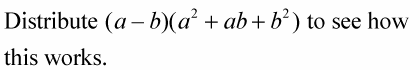1. Distribute the a and the –b over the trinomial.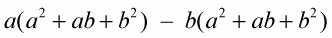2. Distribute the two values separately and multiply each term.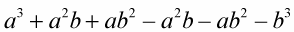3. Combine like terms.

Notice that the four terms in the middle are all pairs of opposites that add up to zero.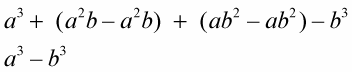This pattern always results in the difference of two cubes.

Example: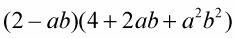You can find the pattern in this example, which has terms comprising known numbers and multiple variables.